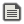# INC vs PUSH vs COPY vs +12Me21Created:S\$=S\$+D\$ is REALLY slow:
```C=MILLISEC
FOR I=1 TO 10000
'code to test
NEXT
?MILLISEC-C```
results: INC S\$,D\$: 16 ms PUSH S\$,D\$: 24 ms COPY S\$,LEN(S\$),D\$: 34 ms S\$=S\$+D\$: 667 ms The reason S\$=S\$+D\$ is so slow is because it needs to make a new string every time, while PUSH INC and COPY just modify the original string.The main problem with those commands, though, is this code:
```A\$="Hello"
B\$=A\$
INC A\$," there"
?B\$
'Hello there
```
B\$ will reference the same memory as A\$, which is usually not what you want. A simple solution to that would be replacing line 2 with
`B\$=A\$+"" 'Empty string, so it makes a new copy`The main problem with those commands
What does this have to do with what 12 is talking about?The main problem with those commands
What does this have to do with what 12 is talking about?
Just saying that when people use those commands to speed up their program, it's worth taking note some of the consequences.If you're going to create a new string, you might as well add " world" right away
```A\$="Hello"
B\$=A\$+" there"
?B\$```True. The solution more applies to a situation like this:
```A\$="Hello"
B\$=A\$+""
FOR I=1 TO 100
INC B\$,CHR\$(RND(26)+65)
NEXT
?B\$```
I don't know where that particular code block would be useful, but something similar.I just thought of a possibly better alternative to B\$=A\$+"": B\$=A\$*1 I'm not sure if it's faster, but it's shorter and I think it looks better.It does look a lot better. Doing a speed test nowThis is why I call \$ the pointer type in Lowerdash... This behavior is also why the delete command even works in my stupid language ;)```C=MILLISEC
A\$="Hello"
FOR I=1 TO 10000
'CODE
NEXT
?MILLISEC-C```
Speeds: B\$=A\$+"Y" 20 milliseconds B\$=A\$+"" 19 milliseconds A\$=A\$+"" 19 milliseconds A\$=A\$+"Y" 222 milliseconds A\$=A\$*1 18 milliseconds B\$=A\$*1 18 milliseconds It seems to be a *little* bit faster As in it saves you 100 nanoseconds, or 1/10000 millisecondIt's also weird that there is such a large difference between A\$=A\$+"Y" and B\$=A\$+"Y"A\$=A\$+"Y" is slow because the string gets really long. EDIT: also usually the results vary by 1 or 2 milliseconds each time, so *1 might not actually be any faster. And if you're getting numbers less than 100, I recommend that you add another 0 to the FOR loop so it runs for 10 times longer.```DIM AV1,AV2
A\$="HELLO"
FOR J=0 TO 199
C=MILLESEC
FOR I=0 TO 100000
B\$=A\$*1
NEXT
PUSH AV1,MILLESEC-C
NEXT
FOR J=0 TO 199
C=MILLESEC
FOR I=0 TO 100000
B\$=A\$+""
NEXT
PUSH AV2,MILLESEC-C
NEXT
?AVERAGE(AV1)
?AVERAGE(AV2)

COMMON DEF AVERAGE(A[])
S=0
FOR I=0 TO LEN(A)-1
INC S,A[I]
NEXT
RETURN S/LEN(A)
END
```
Results for 2 runs: *1=195.84,195.84 +""=196.005,196.36 Results from tacking on an extra zero:(took absolutely forever to run, even on new 3ds) *1=1956.385 +""=1961.905 Basically means you can save 5 milliseconds after 400,000,000. So they might as well be the same thing